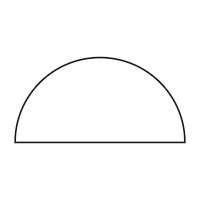# Program to find the Area and Perimeter of a Semicircle in C++

In this problem, we are given a value that denotes the radius of a semicircle. Our task is to create a program to find the Area and Perimeter of a Semicircle in C++.

SemiCircle is a closed figure that is half of a circle.Let’s take an example to understand the problem,

## Input

R = 5

## Output

area = 39.25 perimeter = 15.7

## Solution Approach

To solve the problem, we will use the mathematical formula for the area and perimeter of a semi-circle which is derived by dividing the area of circle by 2.

Area of semicircle,A= $½(\prod^*a^2)=1.571^*a^2$

Perimeter of semicircle, P =(π*a)

Area of semicircle, area = $½(π^*a^2)$

Program to illustrate the working of our solution

## Example

Live Demo

#include <iostream>
using namespace std;
float calaAreaSemi(float R) {
return (1.571 * R * R);
}
float calaPeriSemi(float R) {
return (3.142 * R);
}
int main(){
float R = 5;
cout<<"The radius of semicircle is "<<R<<endl;
cout<<"The area of semicircle is "<<calaAreaSemi(R)<<endl;
cout<<"The perimeter of semicircle is "<<calaPeriSemi(R)<<endl;
return 0;
}

## Output

The radius of semicircle is 5
The area of semicircle is 39.275
The perimeter of semicircle is 15.71